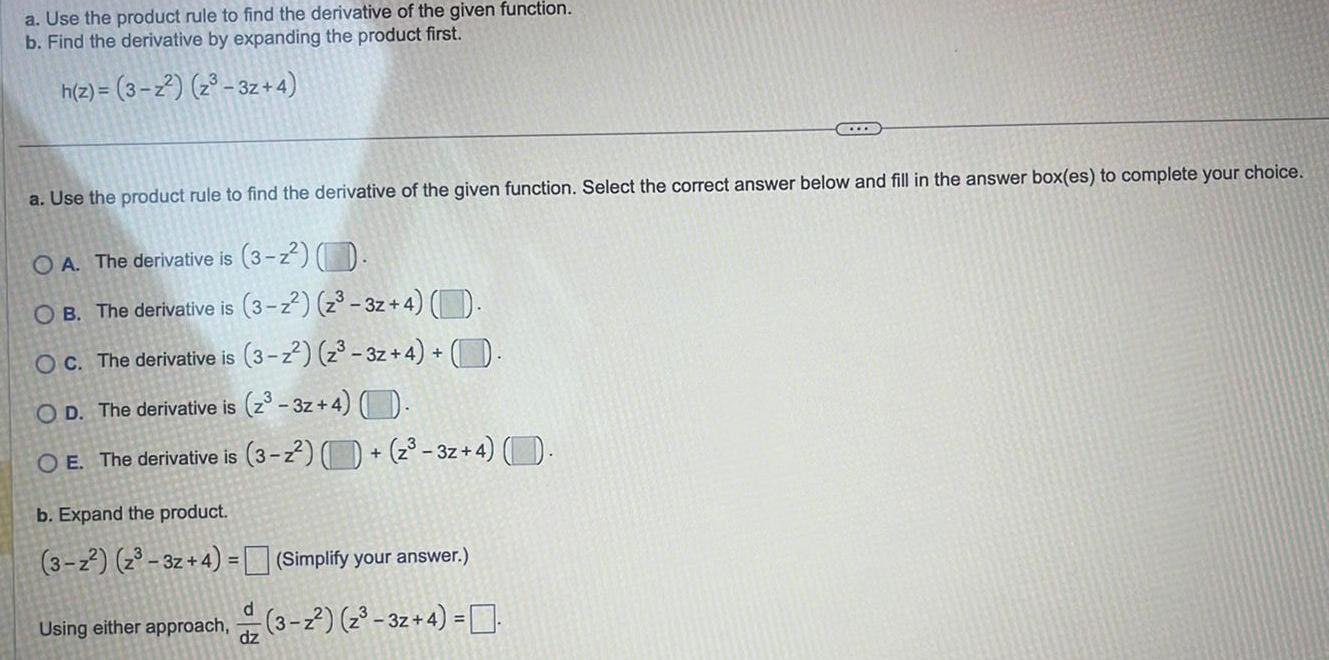Question:

# a Use the product rule to find the derivative of the given

Last updated: 9/16/2023a Use the product rule to find the derivative of the given function b Find the derivative by expanding the product first h z 3 2 3z 4 a Use the product rule to find the derivative of the given function Select the correct answer below and fill in the answer box es to complete your choice OA The derivative is 3 z O B The derivative is 3 z z 3z 4 OC The derivative is 3 z z 3z 4 OD The derivative is 2 3z 4 The derivative is 3 z z 3z 4 b Expand the product 3 2 z 3z 4 Using either approach dz TILB Simplify your answer 3 z z 3z 4